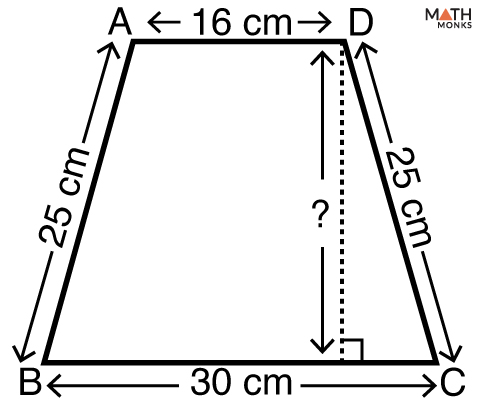# How to Find the Height of a Trapezoid

## Height of a Trapezoid

The height (altitude) of a trapezoid is the distance between its two bases (long base and short base). It is always perpendicular to either of the bases.

This article will precisely deal with how to how to find the height of a trapezoid.

## Formula

### How to Find the Height of a Trapezoid With Area and Bases

We can use the basic formula of the area of a trapezoid and calculate its height when its area and bases are known.

The basic formula to find the area is

Area (A) = ½ (a + b) × h, here a = long base, b = short base, h = height

Now, h = 2A/(a + b)

Let us solve an example to understand the concept.

Find the height of a trapezoid with area 364 cm2 whose bases are 15 cm and 11 cm.

Solution:

As we know,
Height (h) = 2A/(a + b), here A = 364 cm2, a = 15 cm, b = 11 cm
∴h = 2 × 364/(15 + 11)
= 28 cm

### How to Find the Height of a Trapezoid Without Area

When we know the bases and legs of an isosceles trapezoid, we can find the height even when the area is not given.

Let us solve an example to understand the concept.Find the height of an isosceles trapezoid with bases 30 cm and 16 cm, and legs 25 cm each.Solution:

In trapezoid ABCD,
Here BC = 30 cm, AD = 16 cm
Let PC = x
Since, it is an isosceles trapezoid,
∴ h = $(\sqrt{25^{2}-7^{2}})$